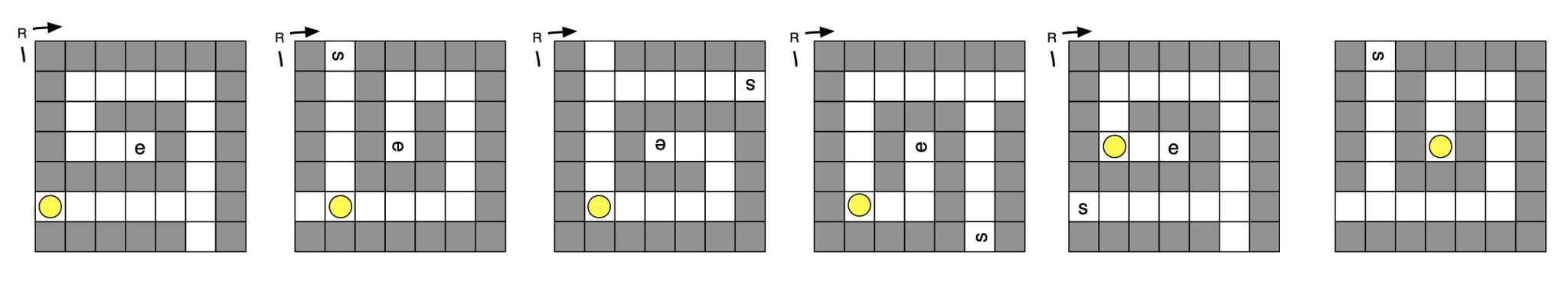시간 제한 메모리 제한 제출 정답 맞은 사람 정답 비율
1 초 128 MB 0 0 0 0.000%

## 문제

Kevin Flynn (Sam’s father) likes to design innovative and fun video games for his video arcade. He is currently thinking about a maze game with a twist (literally) as discussed below. Since not all mazes are solvable, you are to help him decide whether a particular maze is solvable or not.

In the game, you are provided a maze, specified by a 2D array of cells. Each cell can be either empty, a wall, the starting point, or the ending point. A ball starts at the cell marked as the starting point and your goal is to get the ball to the end point. Gravity is acting in the maze and the ball will fall down the screen unless a wall is supporting it from below.

The operations you can perform are to either (a) Rotate the maze 90 to the left (counter-clockwise), or (b) Rotate the maze 90◦ to the right (clockwise). A solution is a string of of “L” and “R”, indicating a series of left and right rotations that will cause the ball to come to rest at the designated ending point. If no solution exists, return the string “NONE”.

For example, consider the input maze at the far left:The solution here is “RRRRR” – i.e., a sequence of 5 clockwise rotations will bring the ball to rest at the ending point.

Here are some more specific details about the game.

1. The ball can pass through the starting and ending point. It needs to come to rest on the ending point for it to be a valid solution. For example, if the ending point was the empty square adjacent to the current ending point in the above example, there is no solution since the ball will always pass through that ending point.
2. The start and end points do not need to be at the edge of the board.
3. The maze does not have to be completely enclosed. It is possible for the ball to fall off the board.
4. If there is more than one sequence of rotations, return the shortest one. If there are several shortest sequences, return the one that would come alphabetically first if viewed as a word.
5. The board starts in the orientation as it is shown in the file (with gravity acting down the screen). The ball should be allowed to fall from its starting place (if it can) before any rotations occur.

## 입력

The first line of test data file contains the number of test cases (≤ 20). Then the input cases are listed one after the other. Each input case looks like this:

nx ny
XXXXXXXXXXX
XsX...X...X
X.X.X.X.X.X
X.X.X.X.X.X
X...X...XeX
XXXXXXXXXXX

where nx and ny are integers giving the number of columns and rows in the maze, respectively. Following these numbers are ny lines, each of length nx. Each character in these lines represents a cell of the maze. A “.” character represents an empty part of the maze (over which the ball can roll). The “s” and “e” characters represent the starting and goal (end) positions of the ball, respectively. Leading and trailing whitespace is removed. Any other character represents an obstacle (a wall) that the ball cannot pass through.

## 출력

Return a string that lists out the sequence of turns to achieve the goal. If there is more than one sequence of rotations, return the shortest one. If there are several shortest sequences, return the one that would come alphabetically first if viewed as a word. If there is no way to solve the maze, then return “NONE”.

## 예제 입력 1

3
4 4
xxxx
xs.x
x.ex
xxxx
13 6
XXXXXXXXXXXXX
X...X...X...X
X.X.X.X.X.X.X
X.X.X.X.X.X.X
XsX...X...XeX
XXXXXXXXXXXXX
13 6
XXXXXXXXXXXXX
X...X...X....
X.X.X.X.X.X.X
X.X.X.X.X.X.X
XsX...X...XeX
XXXXXXXXXXXXX


## 예제 출력 1

Case 1: R
Case 2: LLLLRRLLRRLL
Case 3: NONE